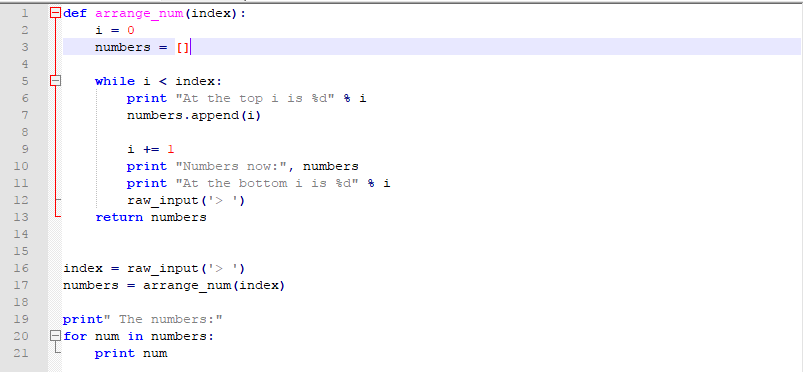Python下的while循环问题求教4个回答

1、假如你第一次输入一个数index=10，首先while那里你要转int(index)才行，要不然报错。然而你里面的input是没有啥作用的，仅仅等着用户输入后执行下次循环，并没有改变index值。所以当i自增到index后就能跳出去了，你要是输入个10000那你就得循环10000次才跳出去了，你input一次它走一次循环。
2、改index=input()可以。

def arrange_num(index):
i = 0
numbers = []

``````while i< index:
print "At the top i is %d" % i
numbers.append(i)

i += 1
print "Numbers now:", numbers
print "At the bottom i is %d" % i
#raw_input('>')
return numbers
``````

index = raw_input('>')
numbers = arrange_num(int(index))

print "The numbers:"
for num in numbers:
print num